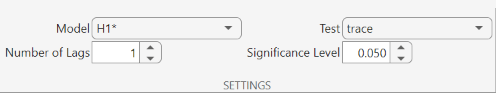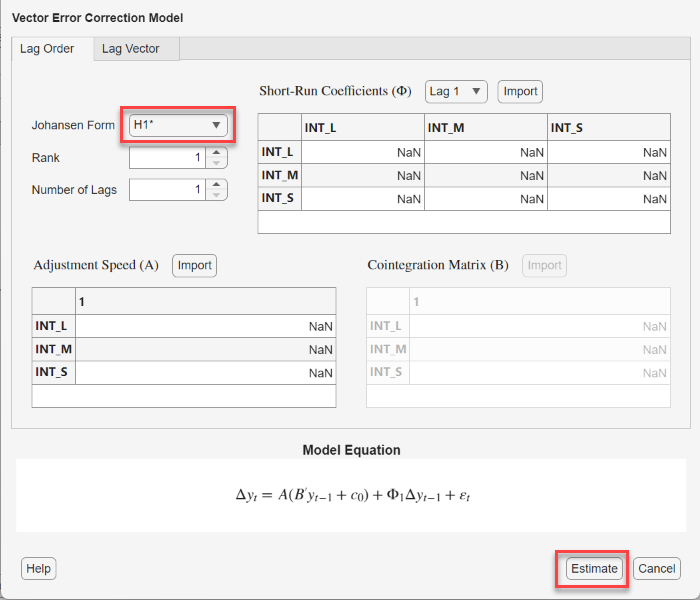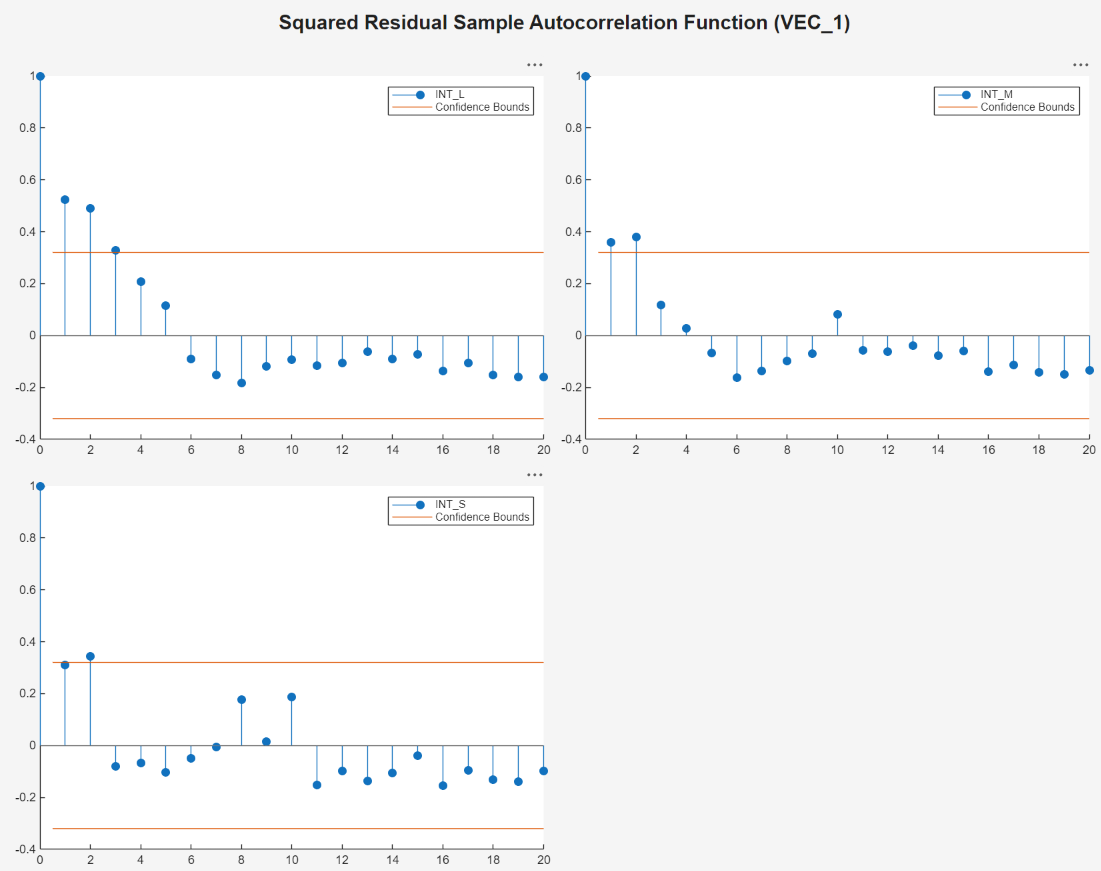## Estimate Vector Error-Correction Model Using Econometric Modeler App

This example models the annual Canadian inflation and interest rate series by using the Econometric Modeler app. The example performs the following actions in the app:

1. Test each raw series for stationary.

2. Test for cointegration and determine a Johansen cointegration form if cointegration is present.

3. Fit several completing vector error-correction (VEC) models, and choose the one with the best, parsimonious fit.

4. Diagnose each residual series.

5. Export the chosen model to the command line.

At the command line, the example uses the model to generate forecasts.

The data set, which is stored in `Data_Canada`, contains annual Canadian inflation and interest rates from 1954 through 1994.

### Load and Import Data into Econometric Modeler

At the command line, load the `Data_Canada.mat` data set.

`load Data_Canada`

Convert the table `DataTable` to a timetable:

1. Clear the row names of `DataTable`.

2. Convert the sampling years to a `datetime` vector.

3. Convert the table to a timetable by associating the rows with the sampling times in `dates`.

```DataTable.Properties.RowNames = {}; dates = datetime(dates,12,31,'Format','yyyy'); DataTable = table2timetable(DataTable,'RowTimes',dates);```

At the command line, open the Econometric Modeler app.

`econometricModeler`

Alternatively, open the app from the apps gallery (see Econometric Modeler).

Import `DataTable` into the app:

1. On the Econometric Modeler tab, in the Import section, click.

2. In the Import Data dialog box, in the Import? column, select the check box for the `DataTable` variable.

3. Click .

The Canadian interest and inflation rate variables appear in the Time Series pane, and a time series plot of all the series appears in the Time Series Plot(INF_C) figure window.Plot only the three interest rate series `INT_L`, `INT_M`, and `INT_S` together. In the Time Series pane, click `INT_L` and Ctrl click `INT_M` and `INT_S`. Then, on the Plots tab, in the Plots section, click Time Series. The time series plot appears in the Time Series(INT_L) document.The interest rate series each appear nonstationary, and they appear to move together with mean-reverting spread, in other words, they exhibit cointegration. To establish these properties, this example conducts statistical tests.

### Conduct Stationarity Test

Assess whether each interest rate series is stationary by conducting Phillips-Perron unit-root tests. For each series, assume a stationary AR(1) process with drift for the alternative hypothesis (you can confirm this property by viewing the autocorrelation and partial autocorrelation function plots).

Close all plots in the right pane, and perform the following procedure for each series `INT_L`, `INT_M`, and `INT_S`.

1. In the Time Series pane, click a series.

2. On the Econometric Modeler tab, in the Tests section, click > Phillips-Perron Test.

3. On the PP tab, in the Parameters section, in the Number of Lags box, type `1`, and in the Model list select Autoregressive with Drift.4. In the Tests section, click . Test results for the selected series appear in the PP(`series`) tab.

Position the test results to view them simultaneously.All tests fail to reject the null hypothesis that the series contains a unit root process.

### Conduct Cointegration Test

In order to create and estimate a VEC model of unit root series, the series need to exhibit cointegration. Conduct a Johansen test. Because the raw series do not contain a linear trend, assume that the only deterministic term in the model is an intercept in the cointegrating relation (`H1*` Johansen form), and include 1 lagged difference term in the model.

1. In the Time Series pane, click `INT_L` and Ctrl click `INT_M` and `INT_S`.

2. In the Tests section, click > Johansen Test.

3. On the JCI tab, in the Parameters section, in the Number of Lags box, type `1`, and in the Model list select H1*.4. In the Tests section, click . Test results and a plot of the cointegration relation for the largest rank appear in the JCI tab.Econometric Modeler conducts a separate test for each cointegration rank 0 through 2 (the number of series – 1). The test rejects the null hypothesis of no cointegration (Cointegration rank = 0), but fails to reject the null hypothesis of Cointegration rank ≤ 1. The conclusion is to set the cointegration rank of the VEC model to 1.

### Estimate VEC Models

Estimate 3-D VEC(`p`) models of the interest rate series, with a cointegration rank of 1 and `p` = 1 and 2.

1. With `INT_L`, `INT_M`, and `INT_S` selected in the Time Series pane, in the Models section, click VEC.

2. In the VEC Model Parameters dialog, in the Johansen Form, select H1*. Fit the VEC(1) model by clicking .The model variable `VEC` appears in the Models pane, its value appears in the Preview pane, and its estimation summary appears in the Model Summary(VEC) document.

3. Repeat step 1 and 2 for the short-run polynomial order `p` = 2.

Similar to the VEC(1) estimation, the variable `VEC2` appears in the appears in the Models pane, and its estimation summary appears in the Model Summary(VEC2) document. You can view properties of an estimated model in the Preview pane by clicking the model in the Models pane. For example, click `VEC`.### Select Model With Best In-Sample Fit

The estimation summary in each Model Summary(VEC`p`) tab contains a plot of fitted values and residuals, with respect to the time series in the Time Series list, a standard statistical table of estimates and inferences, and a table of information criteria.

Compare the information criteria of each estimated model simultaneously by positioning the estimation summary documents so that they occupy the left and right sections of the right pane. The model with the lowest value has the best, parsimonious fit.The VEC(1) model `VEC` produces the lowest AIC and BIC values. Choose this model for further analysis.

### Check Goodness of Fit

Inspect the following VEC(1) plots of each residual series:

• Histograms, for center, normality, and outliers

• Quantile-quantile plots, for normality, skewness, and tails

• Autocorrelation function (ACF), for serial correlation

• ACF of squared residual series, for heteroscedasticity

This example diagnoses the residuals visually. Alternatively, you can conduct statistical tests to diagnose the residuals.

Dismiss the Model Summary(VEC2) document by clickingon its tab.

On the Models pane, click `VEC`.

Plot separate residual histograms. On the Econometric Modeler tab, in the Diagnostics section, click > Residual Histogram. Histograms of the each residual series appear in the Histogram(VEC) document.Each residual series appears approximately centered around 0 and approximately normal.

Plot separate residual quantile-quantile plots. With `VEC` selected in the Time Series pane, on the Econometric Modeler tab, in the Diagnostics section, click > Residual Q-Q Plot. Quantile-quantile plots of the each residual series appear in the QQPlot(VEC) document.The residual series have are slightly skewed left and have lighter tails than the normal distribution. This example proceeds without addressing possible the skewness and light tails.

Plot separate ACF plots of each residual series. With `VEC` selected in the Time Series pane, on the Econometric Modeler tab, in the Diagnostics section, click > Autocorrelation Function. ACF plots of the each residual series appear in the ACF(VEC) document.The residuals do not exhibit significant autocorrelation.

Plot separate ACF plots of each squared residual series. With `VEC` selected in the Time Series pane, on the Econometric Modeler tab, in the Diagnostics section, click > Squared Residual Autocorrelation. ACF plots of the each squared residual series appear in the ACF(VEC)2 document.Each squared residual series has significant autocorrelations at early lags, which suggests that heteroscedasticity is present in all series. This example proceeds without addressing possible heteroscedasticity.

### Export Model to Workspace

Export the model to the workspace.

1. With the `VEC` model selected in the Models pane, on the Econometric Modeler tab, in the Export section, click > .

2. In the Export Variables dialog box, select the Select check box the `INT_L`, `INT_M`, and `INT_S`.

3. Click .

The variables `INT_L`, `INT_M`, `INT_S`, and `VEC` appear in the workspace.

### Generate Forecasts at Command Line

Generate forecasts and approximate 95% forecast intervals from the estimated VEC(1) model for the next five years. For convenience, use the entire series as a presample for the forecasts (`forecast` discards all specified presample observations except for the required final observation).

```Y0 = [INT_L INT_M INT_S]; [YF,YFMSE] = forecast(VEC,5,Y0); YFSE = cell2mat(cellfun(@(x)sqrt(diag(x)'),YFMSE,UniformOutput=false)); UB = YF + 1.96*YFSE; LB = YF - 1.96*YFSE; datesF = DataTable.Time(end) + calyears(1:5); figure tiledlayout(3,1) for j = 1:VEC.NumSeries nexttile h1 = plot(DataTable.Time,Y0(:,j),Color=[.75,.75,.75]); hold on h2 = plot(datesF,YF(:,j),"r",LineWidth=2); h3 = plot(datesF,UB(:,j),"k--",LineWidth=1.5); plot(datesF,LB(:,j),"k--",LineWidth=1.5); ct = [Y0(end,j) YF(1,j); Y0(end,j) LB(1,j); Y0(end,j) UB(1,j);]; plot([DataTable.Time(end); datesF(1)],ct,Color=[.75,.75,.75]) legend([h1 h2 h3],VEC.SeriesNames(j),"Forecast", ... "Forecast Interval",Location="northwest") hold off end```